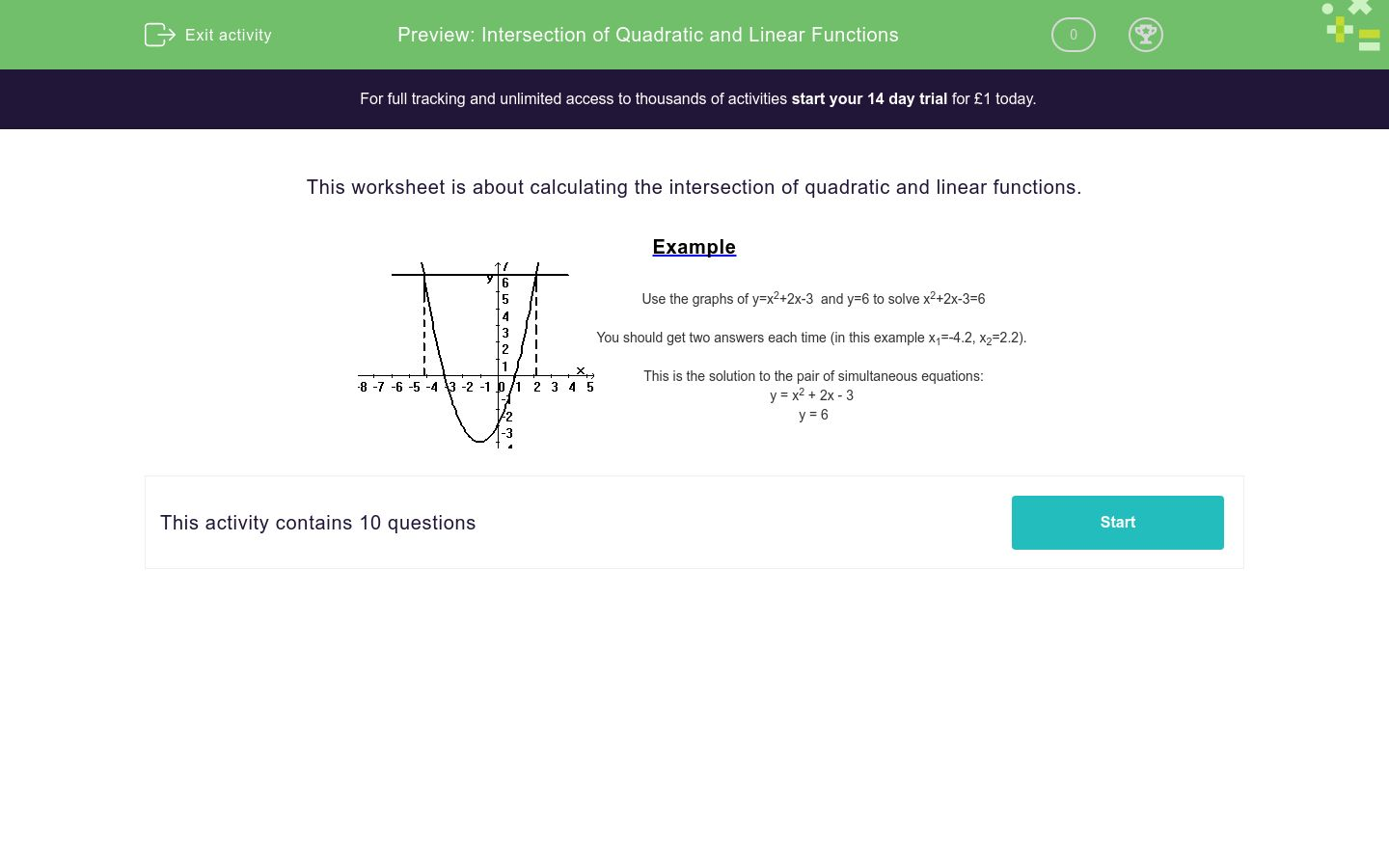# Intersection of Quadratic and Linear Functions

In this worksheet, students find the intersection of quadratic and linear functions using graphs. Graph paper will be needed.Key stage:  KS 4

Curriculum topic:  Algebra

Difficulty level:### QUESTION 1 of 10

This worksheet is about calculating the intersection of quadratic and linear functions.

ExampleUse the graphs of y=x2+2x-3  and y=6 to solve x2+2x-3=6 You should get two answers each time (in this example x1=-4.2, x2=2.2). This is the solution to the pair of simultaneous equations: y = x2 + 2x - 3 y = 6

Solve this pair of simultaneous equations:

y = x2 +8x +7
y=3

x1 =
x2 =

Note that x1 < x2

 x1 x2 -7.5 7.5 0.5 -0.5

Solve this pair of simultaneous equations:

y = x2+2x -7
y=7

x1 =
x2 =

Note that x1 < x2

 x1 x2 -10.2 7.5 2.9 -4.9

Solve this pair of simultaneous equations:

y = x2-7x-7
y=1

x1 =
x2 =

Note that x1 < x2

 x1 x2 -1 7.5 0.5 8

Solve this pair of simultaneous equations:

y = x2 -5x +6
y=2

x1 =
x2 =

Note that x1 < x2

 x1 x2 1 2 3 4

Solve this pair of simultaneous equations:

y = x2 -8x +8
y=6

x1 =
x2 =

Note that x1 < x2

 x1 x2 0.1 7.7 0.3 7.4

Solve this pair of simultaneous equations:

y = x2 -x -10
y=2

x1 =
x2 =

Note that x1 < x2

 x1 x2 4 7.7 -3 7.4

Solve this pair of simultaneous equations:

y = x2 -6x + 5
y=5

x1 =
x2 =

Note that x1 < x2

 x1 x2 -4 0 -3 6

Solve this pair of simultaneous equations:

y = x2 + 9x - 4
y=4

x1 =
x2 =

Note that x1 < x2

 x1 x2 -4 0.8 -9.8 6

Solve this pair of simultaneous equations:

y = x2 - 2x - 4
y=7

x1 =
x2 =

Note that x1 < x2

 x1 x2 -4 -2.5 -9.8 4.5

Solve this pair of simultaneous equations:

y = x2 + 2x - 3
y=6

x1 =
x2 =

Note that x1 < x2

 x1 x2 -4.2 -2.5 -9.8 2.2
• Question 1

Solve this pair of simultaneous equations:

y = x2 +8x +7
y=3

x1 =
x2 =

Note that x1 < x2

 x1 x2 -7.5 7.5 0.5 -0.5
• Question 2

Solve this pair of simultaneous equations:

y = x2+2x -7
y=7

x1 =
x2 =

Note that x1 < x2

 x1 x2 -10.2 7.5 2.9 -4.9
• Question 3

Solve this pair of simultaneous equations:

y = x2-7x-7
y=1

x1 =
x2 =

Note that x1 < x2

 x1 x2 -1 7.5 0.5 8
• Question 4

Solve this pair of simultaneous equations:

y = x2 -5x +6
y=2

x1 =
x2 =

Note that x1 < x2

 x1 x2 1 2 3 4
• Question 5

Solve this pair of simultaneous equations:

y = x2 -8x +8
y=6

x1 =
x2 =

Note that x1 < x2

 x1 x2 0.1 7.7 0.3 7.4
• Question 6

Solve this pair of simultaneous equations:

y = x2 -x -10
y=2

x1 =
x2 =

Note that x1 < x2

 x1 x2 4 7.7 -3 7.4
• Question 7

Solve this pair of simultaneous equations:

y = x2 -6x + 5
y=5

x1 =
x2 =

Note that x1 < x2

 x1 x2 -4 0 -3 6
• Question 8

Solve this pair of simultaneous equations:

y = x2 + 9x - 4
y=4

x1 =
x2 =

Note that x1 < x2

 x1 x2 -4 0.8 -9.8 6
• Question 9

Solve this pair of simultaneous equations:

y = x2 - 2x - 4
y=7

x1 =
x2 =

Note that x1 < x2

 x1 x2 -4 -2.5 -9.8 4.5
• Question 10

Solve this pair of simultaneous equations:

y = x2 + 2x - 3
y=6

x1 =
x2 =

Note that x1 < x2